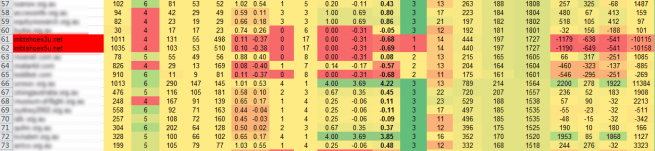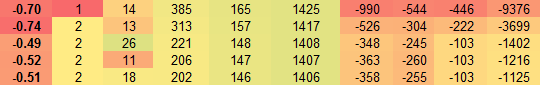In this article we describe quantitative metric-based link spam detection in a collection of analysed domains. Accuracy and co-relation ranges from 60 to 90 percent with adjustment of different quality parameters. Our technique is still considered to be ‘work in progress’ and may not be fit for accurate automated action. Instead we offer a rudimentary, top-level analysis method, suitable for first round flagging and creation of spam alerts within large collections of domains and massive backlink profiles.In the above image we show the case of strong co-relation with the metrics on the right and the manually flagged spam domains showing in red on the left.

## Metrics & Calculation

Although not all metrics proved to be useful in our analysis we will list all included in our spreadsheet (from left to right starting with column A and ending with W):

1. Domain
4. PageRank Sum (tPR)
5. Unique PageRank Sum (uPR)
6. Unique Domain Backlink Sum (udBL)
7. D-Factor (D=uPR/udPR)
8. Average D-Factor Difference (avgD=D-(D1+Dn…/n)
9. Unique Government Domain Links (uGOV)
10. Unique Educational Domain Links (uEDU)
11. Trusted D-Factor (tD=uGOV/uEDU)
12. Average Trusted D-Factor (avgtD=tD-(tD1+tDn…/n)
14. Manual Trust Value (mT=Manually entered for benchmarking purposes)
15. Domain Length (L)
16. Formula Variants:
1. S1(n10)=((F2*E2)/100+(J2*10)+(I2*10))*N2
2. S2(n10v)=((F2*E2)/W2+(J2*10)+(I2*10))*N2
3. S3(n100v)=((F2*E2)/(W2)+(J2*100)+(I2*100))*N2
4. S4(m)=((F2*E2)/(W2)+(J2*100)+(I2*100))*M2
5. S5(h)=((F2*E2)/(W2)+(J2*100)+(I2*100))*H2
6. S6(l)=((F2*E2)/(W2)+(J2*100)+(I2*100))*L2
7. Sx=(E2*F2)*H2
17. Booster (Arbitrary value of 1000, used for smoothing the detection highlighting gradient and can be adjusted at free will)

## Preliminary Results

The most accurate results were achieved through following formulas:

• S4(m)
• S5(h)
• S6(l)

Simplified formula (Sx) is considered to be a borderline case, however it does offer a massively simplified method of link spam detection which proves to be useful even with considerably degraded result accuracy. The logic behind the simplified formula is as follows: (uPR*udBL)*D.

Likewise aT metric gives reasonable accuracy measure on its own. In the table below the first two rows are accurate guesses (aT values of -0.70 and -0.74) with the other three being false positives. We therefore predict that the aT threshold value in this particular collection is above -0.60, which in our collection of observed domains accurately separated the spam from genuine websites.

As a reminder aT is a sum of Average D-Factor and Average Trusted D-Factor, this means that introduction of edu and gov domains does indeed hint at quality, though we give .gov more weight after observing more than 100 flagged results. Even though .gov domains seem to be better moderated and harder to infiltrate by spammers they are not entirely immune to manipulation (e.g. spam of the public log files and statistics). This is where qualitative analysis comes in, however this is outside of the scope of our study.In the table above, last four columns illustrate our more complex formulas which tend to place a wider gap between the domains with organic and inorganic links.